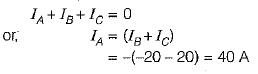Courses

# PolyPhase AC Circuit

## 10 Questions MCQ Test Topicwise Question Bank for GATE Electrical Engineering | PolyPhase AC Circuit

Description
This mock test of PolyPhase AC Circuit for Electrical Engineering (EE) helps you for every Electrical Engineering (EE) entrance exam. This contains 10 Multiple Choice Questions for Electrical Engineering (EE) PolyPhase AC Circuit (mcq) to study with solutions a complete question bank. The solved questions answers in this PolyPhase AC Circuit quiz give you a good mix of easy questions and tough questions. Electrical Engineering (EE) students definitely take this PolyPhase AC Circuit exercise for a better result in the exam. You can find other PolyPhase AC Circuit extra questions, long questions & short questions for Electrical Engineering (EE) on EduRev as well by searching above.
QUESTION: 1

Solution:
QUESTION: 2

### In a balanced three-phase star connected system, the phase voltages

Solution: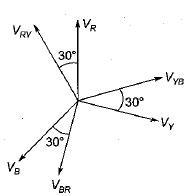Here, line-to-line voltage leads the respective phase voltage by 30°.

QUESTION: 3

### Three identical resistances are connected in delta against a balanced three-phase voltage supply. If one of the resistances be removed, the reduction in the power will be

Solution:

When all the resistance are present,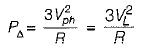(Since Vph= VL for Δ connection)
When one of the phase resistance is removed,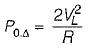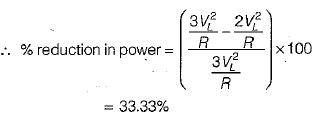QUESTION: 4

In a delta connected system, the line current are

Solution: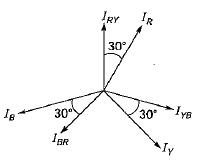QUESTION: 5

A balanced Y-connected load is supplied from a balanced 3-phase 400 V system. If the current in each phase is 15 A and lags the phase voltage by 30°, the total power will be

Solution: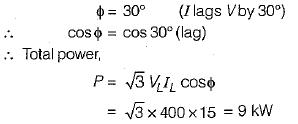QUESTION: 6

In a balanced three-phase circuit VAN= 1500 ∠20° V and VCN = 1500 ∠-100° V.

The value of VBN will be

Solution:

Since VCN lags VAN by 120°, therefore phase sequence will be ACB.
Here, VBN will lead VAN  by 120° but, will have the same magnitude.

so,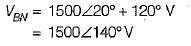QUESTION: 7

The value of the rms line voltage VL for the below circuit if the rms line voltage is 100 V will be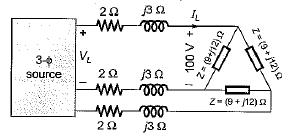Solution:

Converting the Δ-connected load in star equivalent, we have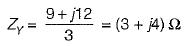So, line to neutral voltage for equivalent Y-load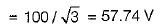∴ Line current,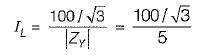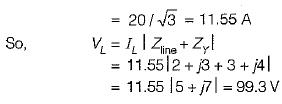∴ Line voltage at source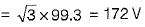QUESTION: 8

Two wattmeters, both have reading of 3 kW when connected for the two-wattmeter method with current coils in lines A and B of a 600 V ABC circuit having a balanced A load. The Δ phase impedance will be

Solution:

we have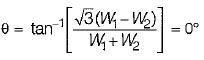(Since W1 = W2 = 3 kW)
or, cosθ= 1

Hence, the load will be purely resistive.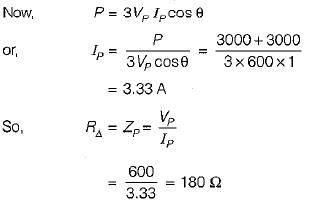QUESTION: 9

Three capacitances, each of C/3 Farads are connected in delta. Their equivalent star value for each capacitance is

Solution:

We know that,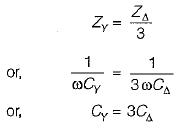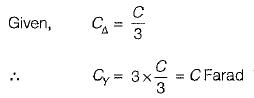QUESTION: 10

The instantaneous value of currents in both phases B and C of a 3-phase balanced system are -20 A. For a phase sequence of ABC, the instantaneous value of current in phase A is

Solution:

Since the system is balanced, therefore